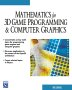# Maths - Square Root of Matrix

Since the square root of a general matrix is difficult we will first try some simpler cases:

## Root of Diagonal Matrix

A diagonal matrix is a matrix where the terms which are not on the leading diagonal are zero.

In this case the result is easy, we just take the roots of the individual diagonal terms.

square root of:
 a 0 0 0 b 0 0 0 c
=
 √a 0 0 0 √b 0 0 0 √c

## Root of Symmetrical Matrix

A symmetrical matrix is one where the terms are symmetrical about the diagonal axis, that is the element xij has the same value as the element xji

For a symmetrical matrix we can rotate it to get a diagonal matrix, then take the root of the diagonal matrix as above, then rotate it back to its original coordinates. This rotation matrix is the eigen matrix or the orthonormal basis of [A], in other words:

[D] = [Q]-1 [A] [Q]

where:

• [D] = Diagonal matrix, diagonal terms are eigenvectors of A
• [A] = Symmetrical Matrix
• [Q] = Orthogonal matrix, columns are eigenvectors of A
• [Q]-1 = inverse of [Q]

So to find the square root of [A] we use:

√[A] = [Q] √[D] [Q]-1

### Example

We want to find the square root of:

 1 0 0 0 1 0 0 0 1

#### Program

There are a number of open source computer algebra programs. I have used Axiom, how to install Axiom here.

I have put user input in red:

 ```(1) -> m := matrix[[1,0,0],[0,1,0],[0,0,1]] +1 0 0+ | | (1) |0 1 0| | | +0 0 1+ Type: Matrix(Integer) (2) -> eigenvalues(m) (2)  Type: List(Union(Fraction(Polynomial(Integer)), SuchThat(Symbol,Polynomial(Integer)))) (3) -> eigenvectors(m) +0+ +0+ +1+ | | | | | | (3) [[eigval= 1,eigmult= 3,eigvec= [|0|,|1|,|0|]]] | | | | | | +1+ +0+ +0+ Type: List(Record(eigval: Union(Fraction(Polynomial(Integer)), SuchThat(Symbol,Polynomial(Integer))), eigmult: NonNegativeInteger,eigvec: List(Matrix(Fraction(Polynomial(Integer)))))) (4) -> eigenMatrix(m) +1 0 0+ | | (4) |0 1 0| | | +0 0 1+ Type: Union(Matrix(Expression(Integer)),...) (5) -> ```

So in this example

√[A] = [Q] √[D] [Q]-1

square root of:
 1 0 0 0 1 0 0 0 1
=
 1 0 0 0 1 0 0 0 1
 √1 0 0 0 √1 0 0 0 √1
 1 0 0 0 1 0 0 0 1
which gives :
 1 0 0 0 1 0 0 0 1
or
 -1 0 0 0 -1 0 0 0 -1

since √1 = ±1

However this method does not seem to find other possible roots such as :

 0 0 1 0 1 0 1 0 0
or
 0 0 -1 0 -1 0 -1 0 0

These also square to the required value as we can see here:

 ```(5) -> a := matrix[[0,0,1],[0,1,0],[1,0,0]] +0 0 1+ | | (5) |0 1 0| | | +1 0 0+ Type: Matrix(Integer) (6) -> a*a +1 0 0+ | | (6) |0 1 0| | | +0 0 1+ Type: Matrix(Integer) (7) -> ```

So, in this case, we can reoder the eigenvectors to form different eigenmatrix but not all orders are roots, for example this does not square to the required value:

 ```(7) -> b := matrix[[0,0,1],[1,0,0],[0,1,0]] +0 0 1+ | | (7) |1 0 0| | | +0 1 0+ Type: Matrix(Integer) (8) -> b*b +0 1 0+ | | (8) |0 0 1| | | +1 0 0+ Type: Matrix(Integer) (9) -> ```

## Root of General Matrix

I have not worked out how to do this yet.

 metadata block see also: Correspondence about this page Book Shop - Further reading. Where I can, I have put links to Amazon for books that are relevant to the subject, click on the appropriate country flag to get more details of the book or to buy it from them.Mathematics for 3D game Programming - Includes introduction to Vectors, Matrices, Transforms and Trigonometry. (But no euler angles or quaternions). Also includes ray tracing and some linear & rotational physics also collision detection (but not collision response). Other Math Books Specific to this page here:

This site may have errors. Don't use for critical systems.